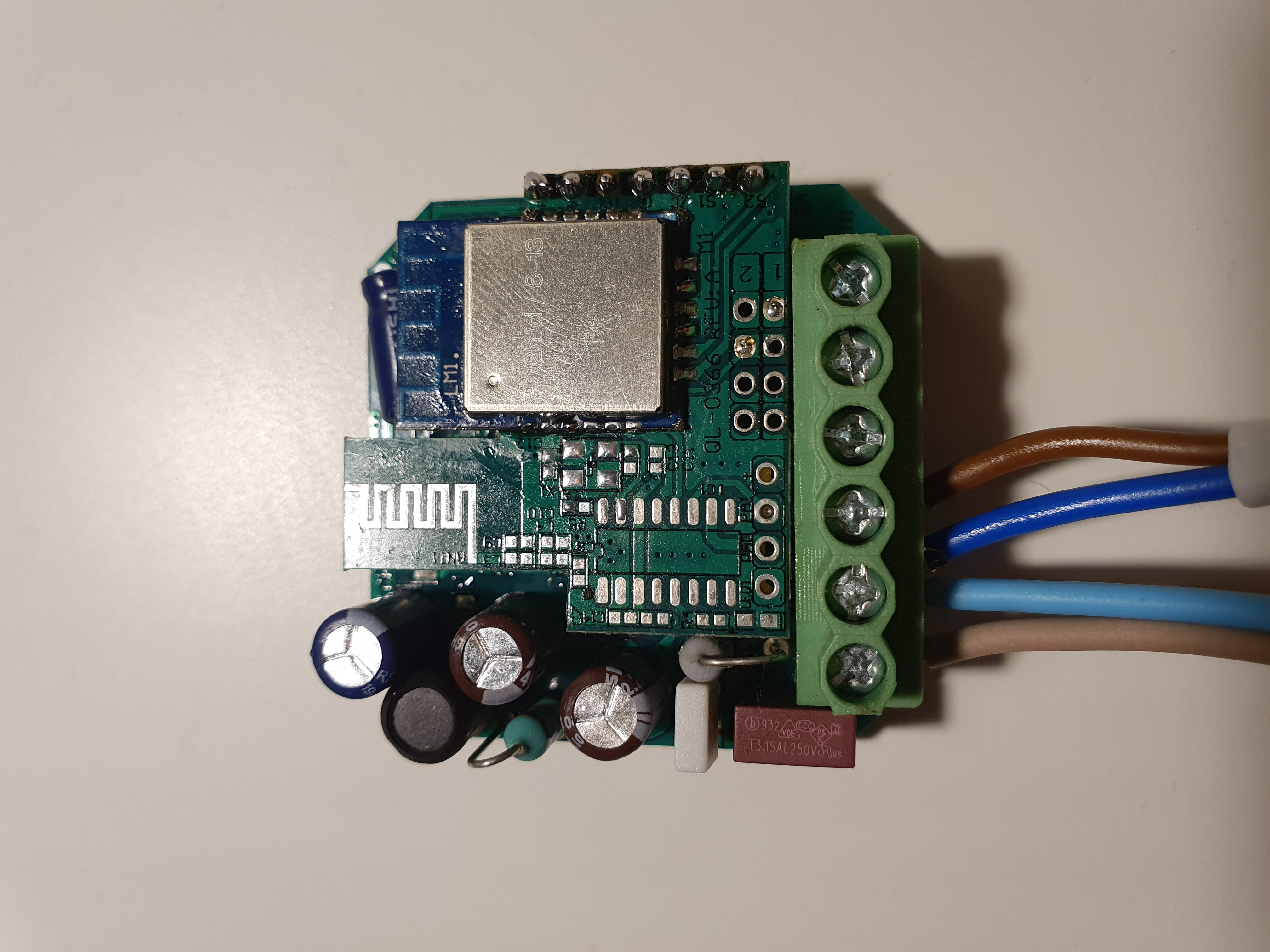Instantly share code, notes, and snippets.

# thxthx0/WiFi-2way-Dimmer-QS-WiFi-D02-Script.txt

Last active November 26, 2021 17:21
Tasmota Script for WiFi-2Channel-Dimmer QS-WiFi-D02-2C or MS-105B-220
This file contains bidirectional Unicode text that may be interpreted or compiled differently than what appears below. To review, open the file in an editor that reveals hidden Unicode characters. Learn more about bidirectional Unicode characters
 ;WiFi-2CH-Dimmer v1.3 ;QS-WiFi-D02-2C ;MS-105B >D sw1=0 sw2=0 x=0 cn1=0 cn2=0 tm1=0 tm2=0 h1=0 h2=0 t1=0 t2=0 sl1=0 sl2=0 di="" pl=2 pu=10 dd1=0 dd2=0 mp=2.2 sp=2 ll=15 ul=95 dv1=70 dv2=70 p1=0 p2=0 >B =>Counter1 0 =>Counter2 0 =>Baudrate 9600 =#sd1(dv1) delay(1000) =#sd1(0) =#sd2(0) >F cn1=pc cn2=pc if chg[cn1]>0 then sw1=1 else sw1=0 endif if chg[cn2]>0 then sw2=1 else sw2=0 endif tm1+=1 tm2+=1 if sw1==0 and tm1>pl and tm1pl and tm20 then if h1==0 then dd1^=1 endif if tm1>pu then h1=1 if t1>0 then if dd1>0 then dv1+=sp if dv1>ul then dv1=ul endif =#sd1(dv1) else dv1-=sp if dv10 then if h2==0 then dd2^=1 endif if tm2>pu then h2=1 if t2>0 then if dd2>0 then dv2+=sp if dv2>ul then dv2=ul endif =#sd2(dv2) else dv2-=sp if dv2E sl1=Channel1 if chg[sl1]>0 then if sl1>0 then dv1=sl1 =#sd1(dv1) else t1=0 =#sd1(0) endif endif sl2=Channel2 if chg[sl2]>0 then if sl2>0 then dv2=sl2 =#sd2(dv2) else t2=0 =#sd2(0) endif endif p1=pwr if p1==1 then t1=1 =#sd1(dv1) else t1=0 =#sd1(0) endif p2=pwr if p2==1 then t2=1 =#sd2(dv2) else t2=0 =#sd2(0) endif #sd1(x) di="FF5501"+hn(x*mp)+"0000000A" =>SerialSend5 %di% =>Channel1 %x% #sd2(x) di="FF550200"+hn(x*mp)+"00000A" =>SerialSend5 %di% =>Channel2 %x% #

### maxela commented Sep 1, 2021

There’s not much happening in this thread, but I get notified and usually I try to help.

Which device and which version of the tasmota firmware are you using?

### SimasVejas commented Sep 1, 2021 • edited

Hi, @maxela, thanks for a quick reply!

At first glance it looks like I'm using the same two channel dimmer that is displayed in this thread with LM1 chip but my version might be a bit different. I will attach a photo of the insides when I get back home from work.
I'm not 100% sure but I believe it was 9.5.### aceindy commented Sep 3, 2021

Just for info, i moved to esphome with this 2ch dimmer unit, it works a lot smoother.
https://community.home-assistant.io/t/qs-wifi-d01-triac-dimmer/149811/25?u=aceindy